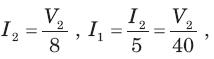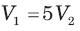Courses

# Magnetically Coupled Circuits - MCQ Test

## 20 Questions MCQ Test GATE ECE (Electronics) 2022 Mock Test Series | Magnetically Coupled Circuits - MCQ Test

Description
This mock test of Magnetically Coupled Circuits - MCQ Test for Railways helps you for every Railways entrance exam. This contains 20 Multiple Choice Questions for Railways Magnetically Coupled Circuits - MCQ Test (mcq) to study with solutions a complete question bank. The solved questions answers in this Magnetically Coupled Circuits - MCQ Test quiz give you a good mix of easy questions and tough questions. Railways students definitely take this Magnetically Coupled Circuits - MCQ Test exercise for a better result in the exam. You can find other Magnetically Coupled Circuits - MCQ Test extra questions, long questions & short questions for Railways on EduRev as well by searching above.
QUESTION: 1

### In the circuit shown in fig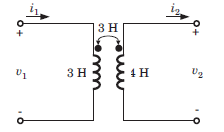i1  = 5sin 3t A and i2 = 3 cos 3t A. v2 = ?

Solution: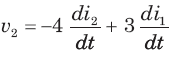=36 sin 3t + 45 cos 3t = 9(4sin 3t + 5cos 3t)  V

QUESTION: 2

### In the circuit shown in fig. if current i1 = 5 cos (500t - 20°) mA and i2 = 20 cos (500t - 20°) mA, the total energy stored in system at t = 0 is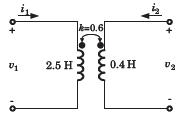Solution: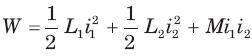At t = 0, i1 = 4 cos (-20°) = 4.7 mA
i2 = 20 cos (-20°) = 18.8 mA,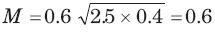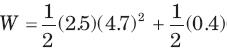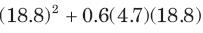= 151.3 μJ

QUESTION: 3

### Leq  = ?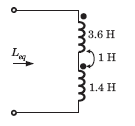Solution: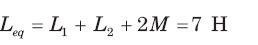QUESTION: 4

Leq = ?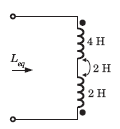Solution: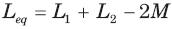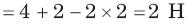QUESTION: 5

Leq = ?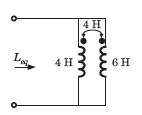Solution: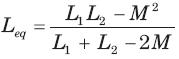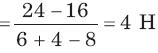QUESTION: 6

Leq = ?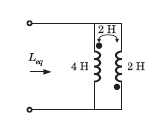Solution: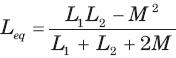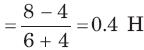QUESTION: 7

The equivalent inductance of a pair of a coupled inductor in various configuration are
(a) 7 H after series adding connection
(b) 1.8 H after series opposing connection
(c) 0.5 H after parallel connection with dotted terminal connected together.

The value of L1 L2 , and M are

Solution: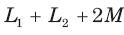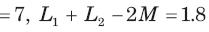⇒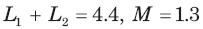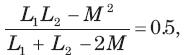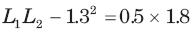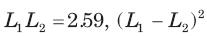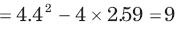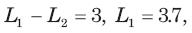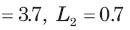QUESTION: 8

Leq = ?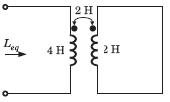Solution: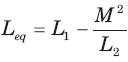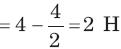QUESTION: 9

Leq = ?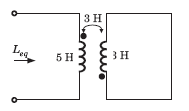Solution: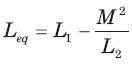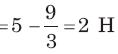QUESTION: 10

Leq = ?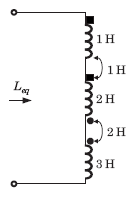Solution: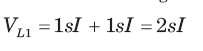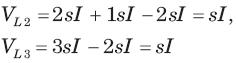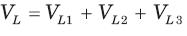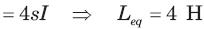QUESTION: 11

Leq = ?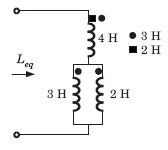Solution:

Let I1 be the current through 4 H inductor and I2 and I3 be the current through 3 H, and 2 H inductor respectively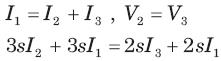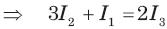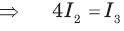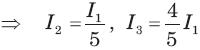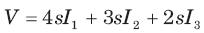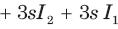QUESTION: 12

Consider the circuit shown in fig.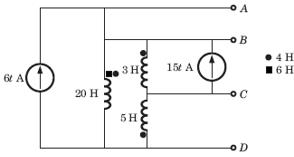The voltage VAG of terminal AD is

Solution: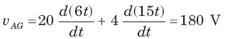QUESTION: 13

Consider the circuit shown in fig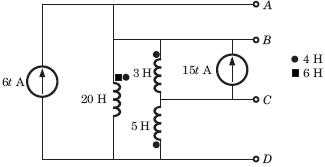The voltage vBG of terminal BD is

Solution: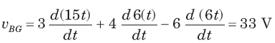QUESTION: 14

Consider the circuit shown in fig.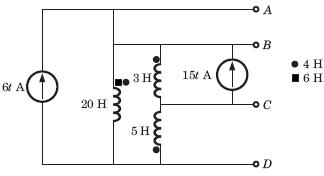The voltage vGC of terminal CD is

Solution: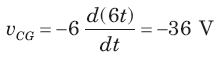QUESTION: 15

In the circuit of fig. the  ω =2 rad/s. The resonance occurs when C is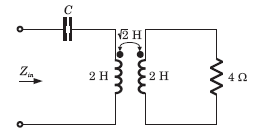Solution: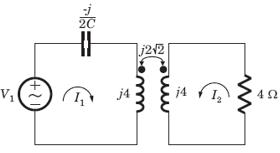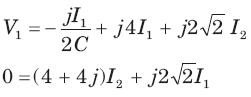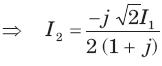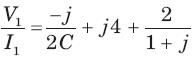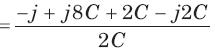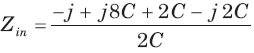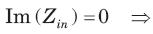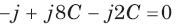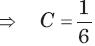QUESTION: 16

In the circuit of fig, the voltage gain is zero at ω= 333.33 rad/s.

The value of C is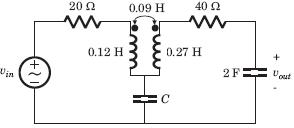Solution: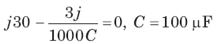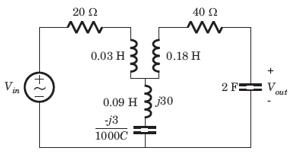QUESTION: 17

In the circuit of fig. at ω = 333.33 rad/s, the voltage gain vout / vin  is zero. The value of C is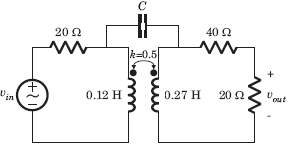Solution:

The π  equivalent circuit of coupled coil is shown in fig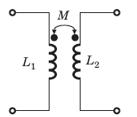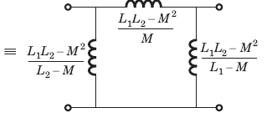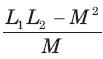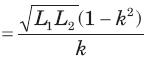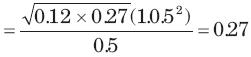Output is zero if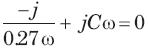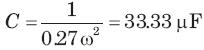QUESTION: 18

The evenin equivalent at terminal ab for the network shown in fig. is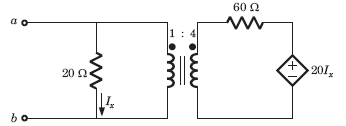Solution:

Applying 1 V test source at ab terminal,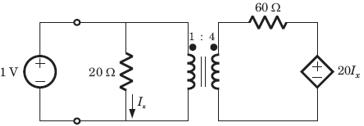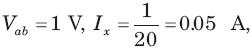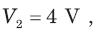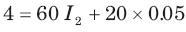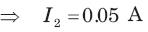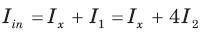= 0.25 A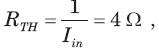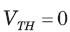QUESTION: 19

In the circuit of fig. the maximum power delivered to RL isSolution:

Impedance seen by RL = 10 x 42 = 160 Ω
For maximum poer RL = 160Ω, Zo = 10Ω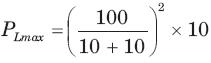= 250 W

QUESTION: 20

The average power delivered to the 8 Ω load in the circuit of fig. is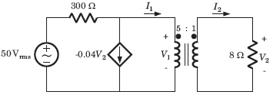Solution: